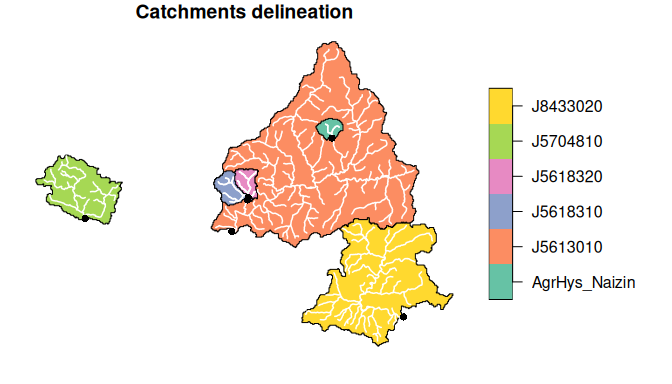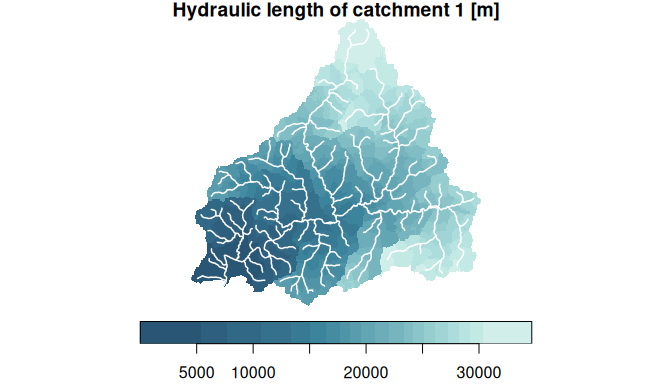# Preparation of input data: geomorphological analysis with whitebox

#### 2023-01-24

The hydrological modelling of the transfR package is based on a geomorphological analysis of the studied catchments. In this vignette, we give some guidance on how to perform this geomorphological analysis. More specifically, we extract the catchment delineation and hydraulic length maps from a digital elevation model (DEM). This analysis is one of the two inputs needed (together with the time series of flow observations) to build a transfR object and start using the transfR package (see the Get started with transfR vignette).

Hydraulic length is defined as the distance within the river network along an identified flow path to the outlet. It can be extracted from a DEM in many different ways, such as with the GRASS toolkits (Jasiewicz and Metz 2011), Whitebox GAT (see J. B. Lindsay (2016) or WhiteboxTools), TauDEM (D. Tarboton, Utah State University) or online services (Squividant et al. (2015) for catchment delineation only). This vignette presents one possible workflow by making use of two main R packages:

Functions of the whitebox package generally do not work with objects in R memory, but with files written on the disk storage. It is therefore advised to define the working directory where these files will be written.

wbt_wd <- tempdir(check = TRUE)

## 1. Retrieve elevation data with elevatr

The elevatr package allows retrieving grids (rasters) of elevation data worldwide for various zoom levels and data sources. It uses the Open Topography Global Datasets API for accessing Shuttle Radar Topography Mission (SRTM) data.

It is necessary to assign a geographic projection for the catchment delineation and hydraulic length maps with the whitebox package. We chose to use the Lambert93 projection, the official projection for maps of metropolitan France, for which the EPSG code is 2154.

library(elevatr)
library(rgdal) # Still often needed by elevatr
library(progress) # Still often needed by elevatr

# Set up a projection (French Lambert-93 projection)
EPSG <- 2154

# Define a bbox that will encompass the catchments of the study area
blavet_bbox <- st_bbox(c(xmin = -3.3, xmax = -2.7, ymax = 48.11, ymin = 47.77),
crs = st_crs(4326))

# Retrieve elevation data as raster
dem_raw <- elevatr::get_elev_raster(st_as_sfc(blavet_bbox), z = 10) # ~76m resolution

# Project and define spatial resolution:
dem_100m  <- st_warp(st_as_stars(dem_raw), cellsize = 100, crs = st_crs(EPSG))
names(dem_100m) <- "warp"

# Set negative values (ocean) to NA
dem_100m[dem_100m < 0] <- NA

# Write to file
write_stars(dem_100m["warp"], file.path(wbt_wd,"dem_100m.tif"))

## 2. Retrieve a known river network and burn it into DEM (optional)

The hydrological modelling distinguish the hillslope from the river network. Both will have very different transfer dynamics, and the transfR package aims to describe the transfer function of the river network only. Defining where this river network begins and the flow path it takes is a key, and non-trivial, issue for hydrogeomorphologists. The easiest way to draw a drainage network from a DEM is usually to define a minimum drainage area threshold at which the drainage network is assumed to start. However, defining this threshold can be difficult as it may vary spatially, especially with the geology of the region. It may therefore be better to use a known river network and force the flow paths to follow it. Here we will use the French TOPAGE river network as a reference (see the description of the Blavet dataset to download it from the Web Feature Service (WFS) “Sandre - Eau France”). We will implement a stream burning technique into the DEM using the function whitebox::wbt_burn_streams_at_roads() and following John B. Lindsay (2016).

library(transfR)
library(whitebox)

# Get the French Topage river network from the Blavet dataset
data(Blavet)
CoursEau_Topage2019 <- Blavet$network # Change projection and write files network_topage <- st_transform(CoursEau_Topage2019, EPSG) st_write(network_topage, file.path(wbt_wd, "network_topage.shp"), delete_layer = TRUE, quiet = TRUE) whitebox::wbt_rasterize_streams("network_topage.shp", base = "dem_100m.tif", output = "network_topage.tif", nodata = 0, wd = wbt_wd) # Burn this river network on the DEM # We will neglect the effect of the road embankments at this DEM resolution of 100m # by creating an empty shapefile for roads st_write(st_sfc(st_multilinestring(),crs = EPSG), file.path(wbt_wd,"roads.shp"), delete_layer = TRUE, quiet = TRUE) whitebox::wbt_burn_streams_at_roads(dem = "dem_100m.tif", streams = "network_topage.shp", roads = "roads.shp", output = "dem_100m_burn.tif", wd = wbt_wd) ## 3. Delineate catchments from their outlets coordinates The whitebox package provides all the tools to perform a usual catchment delineation workflow from a DEM and outlet’s coordinates. It can be done in 6 different steps: # Remove the depressions on the DEM whitebox::wbt_fill_depressions(dem = "dem_100m_burn.tif", output = "dem_fill.tif", wd = wbt_wd) # Flow direction raster whitebox::wbt_d8_pointer(dem = "dem_fill.tif", output = "d8.tif", wd = wbt_wd) # Compute flow accumulation whitebox::wbt_d8_flow_accumulation(input = "d8.tif", pntr = TRUE, output ="facc.tif", wd = wbt_wd) # Extract a stream network (threshold = 1 km2) consistent with flow direction whitebox::wbt_extract_streams(flow_accum = "facc.tif", threshold = 100, # 100 cells for 1 km2 output = "network_1km2.tif", zero_background = TRUE, wd = wbt_wd) whitebox::wbt_remove_short_streams(d8_pntr = "d8.tif", streams = "network_1km2.tif", output = "network_d8.tif", min_length= 200, wd = wbt_wd) Coordinates of the outlets are retrieved from hydro.eaufrance.fr and snapped to the pixel of the river network that is consistent with the previously defined flow directions. # Localize the outlets of the studied catchments (with manual adjustments to help snapping) outlets_coordinates <- data.frame(id = names(Blavet$hl),
X = c(254010.612,255940-100,255903,237201,273672,265550),
Y = c(6772515.474,6776418-200,6776495,6774304-200,6762681,6783313))
outlets <- st_as_sf(outlets_coordinates, coords = c("X", "Y"), crs=2154)
st_write(outlets, dsn = file.path(wbt_wd, "outlets.shp"),
delete_layer = TRUE, quiet = TRUE)

# Snap the outlets on the stream raster
whitebox::wbt_jenson_snap_pour_points(pour_pts = "outlets.shp",
streams = "network_d8.tif",
output = "outlets_snapped.shp",
snap_dist = 200,
wd = wbt_wd)
outlets_snapped <- st_read(file.path(wbt_wd, "outlets_snapped.shp"), quiet = TRUE)

# Delineate catchments
catchments <- st_sfc(crs = EPSG)
for(id in outlets_snapped$id){ st_write(outlets_snapped[outlets_snapped$id==id,],
file.path(wbt_wd, paste0(id, "_outlet.shp")), delete_layer = TRUE, quiet = TRUE)
whitebox::wbt_watershed(d8_pntr = "d8.tif",
pour_pts = paste0(id, "_outlet.shp"),
output = paste0(id, "_catchment.tif"),
wd = wbt_wd)
# Vectorize catchments
contours <- st_contour(drainage, breaks = 1) |> st_geometry() |> st_cast("POLYGON")
contours <- contours[which.max(st_area(contours))]
catchments <- rbind(catchments, st_sf(data.frame(id, geom = contours)))
}

Resulting catchments delineation can be plotted and checked.

# Compare drainage areas to the dataset provided with transfR
compare_areas <- data.frame(
name = names(Blavet$hl), expected_area = st_area(st_geometry(Blavet$obs)) |> units::set_units("km^2") |> round(1),
computed_area = st_area(catchments) |> units::set_units("km^2") |> round(1)
)

print(compare_areas)
#>            name expected_area computed_area
#> 1      J5613010  314.8 [km^2]  314.8 [km^2]
#> 2      J5618310   15.5 [km^2]   15.6 [km^2]
#> 3      J5618320    6.8 [km^2]    6.7 [km^2]
#> 4      J5704810   45.3 [km^2]   45.8 [km^2]
#> 5      J8433020  134.5 [km^2]  134.7 [km^2]
#> 6 AgrHys_Naizin    4.9 [km^2]    5.2 [km^2]
# Plot catchment delineation
par(oma = c(0, 0, 0, 6))
plot(catchments[,"id"], main = "Catchments delineation", key.pos = 4, reset = FALSE)
plot(st_geometry(st_intersection(network_topage,catchments)),
col = "white", lwd = 1.5, add = TRUE)
plot(outlets_snapped, col = "black", pch = 16, add = TRUE)## 4. Compute flow path length and hydraulic length for each catchments

The whitebox package does not provide a function to compute hydraulic length directly. However, it can compute the total downslope flow path length to the outlet using whitebox::wbt_downslope_flowpath_length() function and the downslope distance to the stream using whitebox::wbt_downslope_distance_to_stream() function. The hydraulic length does not take into account the length of the flow path over the hillslopes, so it can be calculated simply by the difference of these two flow distances.

Flow path lengths are computed once for all the study area. For each catchment, this raster is then trimmed (i.e. removing NA values outside the bounding box of the catchment) and the values of flow path lengths are corrected such as the minimum flow path length is equal to half a pixel width/height. The hydraulic lengths are finally gathered in a list as expected by the as_transfr() function.

# Compute hydraulic length
whitebox::wbt_downslope_flowpath_length(d8_pntr = "d8.tif",
output = "fpl.tif",
wd = wbt_wd)
whitebox::wbt_downslope_distance_to_stream(dem = "dem_fill.tif",
streams = "network_topage.tif",
output = "d2s.tif",
wd = wbt_wd)
hl_region <- fpl-d2s
names(hl_region) <- "hl"

# Crop hydraulic length for each catchment
hl <- list()
for(id in catchments$id){ crop <- st_crop(hl_region, catchments[catchments$id==id,])
crop <- crop-min(crop$hl, na.rm = TRUE) crop$hl <- units::set_units(crop$hl, "m") hl[[id]] <- crop } Resulting maps of hydraulic length can be plotted and checked. i <- 1 network <- st_geometry(st_intersection(network_topage,catchments[i,])) plot(hl[[i]], main = paste("Hydraulic length of catchment", i,"[m]"), col = hcl.colors(20, palette = "Teal"), key.pos = 1, reset = FALSE) plot(network, col = "white", lwd = 1.5, add = TRUE)## 5. Creating a transfR object and running a simulation Catchment delineations can be used as the spatial dimension of stars objects to georeference the observed flow time series of gauged catchments and locate ungauged catchments (see vignette Preparation of input data: creation of a stars object for details). Here we will create a stars object using the observed discharge of the Blavet dataset and the delineations that we just computed with whitebox. obs_st <- st_as_stars(list(Qobs = Blavet$obs$Qobs), dimensions = st_dimensions( time = st_get_dimension_values(Blavet$obs,1),
space = st_geometry(catchments)))

The maps of hydraulic length can finally be used to create an object of class transfR by using the function as_transfr() (argument hl) and perform simulations.

obs <- as_transfr(st = obs_st, hl = hl)

A transfer of hydrograph from the gauged catchments to the ungauged catchments can then quickly be implemented using the quick_transfr() function.

obs <- quick_transfr(obs, velocity = "brittany2013", parallel = TRUE, cores = 2, cv = TRUE)

The simulated time series will be available in its stars object as new attributes.

obs\$st